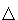# The Taylor Circle

In 1976, Ross Honsberger proposed a problem (#192) in Eureka, a predecessor of Crux Mathematicorum of today (I am grateful to W.McWorter who copied the Eurika's page for me.)

From each of the feet Ha , Hb , Hc of the altitudes ofABC drop a pair of perpendiculars on the other two sides of the triangle. Prove that thus obtained 6 points - Hab , Hba , Hca , Hac , Hbc , Hcb - lie on a circle.As the editor of Eureka commented, the circle was little known at the time (1976). It is still today, as I found it only mentioned once (and that in passing) in a discussion on Conway's circle.

26 February 2015, Created with GeoGebra

The circle is named after H.M.Taylor (1842-1927) who discussed it in 1882. The editor of Eureka remarked further that

In spite of its name, this circle did not originate with Taylor. It was discovered earlier, like so many other good things in life, by the French. It was mentioned by Catalan in 1879, and was apparently first proposed in 1877 by the French mathematician known as Eutaris.

Let H be the orthocenter ofABC. The diagram exhibits a great deal of similar triangles. We start with the following three:

ΔCHaHab ~ ΔCHbHba, ΔCHcHcb ~ ΔCHHb, ΔCHcHca ~ ΔCHHa.

From the similarities, we derive

CHab/CHba = CHa/CHb, CHca/CHc = CHb/CH, CHca/CHc = CHa/CH.

Thus

CHca/CHab = CHa/CHb = CHab/CHba

and

(*)

CHba· CHca = CHab· CHcb

The latter is the converse of Proposition III.35 from Euclid's Elements, namely

If in a circle two straight lines cut one another, then the rectangle contained by the segments of the one equals the rectangle contained by the segments of the other.

(The rectangle in the statement is in fact a rectangle's area, or the product of two segments. This product is the power of the point of intersection with respect to the circle.)

Euclid did not mention this, but the converse to Proposition III.35 is also true:

Let lines DE and FG intersect at a point P. Assume that PD·PE = PF·PG. Then the four points D, E, F, and G lie on a circle.

Assume, on the contrary, that the circle that passes through D, F, and G does not contain E. It then crosses line DE at some point E', for which, as Euclid tells us, PD·PE' = PF·PG.But then PE = PF·PG/PD = PE' so that E = E'. Contradiction.

Back to our original problem, (*) implies that the four points Hba, Hca, Hab, and Hcb lie on a circle. Call it Circlec. Similarly, the four points Hab, Hcb, Hbc, and Hac lie on a circle, say, Circlea, while points Hba, Hca, Hbc, and Hac lie on a circle Circleb.

We would like to show that all three circles coincide. This will be true if any two of them coincide. Thus, let's assume that all three are different. Note that then AB serves as the radical axes of Circlea and Circleb, and the same is true with regard the sides BC and AC and circles Circleb and Circlec and Circlea and Circlec, respectively. As we know, the radical axes of three circles meet at a point, which is absurd unless ΔABC shrinks to a point. Therefore, we conclude that the three circles Circlea, Circleb, and Circlec indeed coincide, so that all six points, Hab , Hba , Hca , Hac , Hbc , Hcb, do lie on a circle.